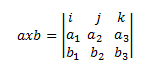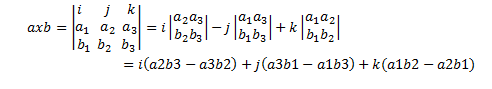# Answer to Question #5959 in Linear Algebra for Lyndsey

Question #5959
find (1,0,0) x (0,0,1)
In this task we need to calculate vector product(or cross product) of two vectors a=(1,0,0) and b=(0,0,1). Recall the definition, if a={a1,a2,a3} and b={b1,b2,b3} then cross product is a vector which is perpendicular to both vectors a={a1,a2,a3} and b={b1,b2,b3} and it&rsquo;s calculated in the following way. We construct determinant out of coordinates of given vectors a and b as shown below:Then appling cofactor expansion we rewrite the determinant opening it by the first row:So axb=(a2*b3 - a3*b2,a3*b1 - a1*b3,a1*b2 - a2*b1).

Hence if a=(1,0,0) and b=(0,0,1) then we have
(1,0,0)x(0,0,1)=(0*1-0*0,-(1*1-0*0),1*0-0*0)=(0,-1,0).

Dear Lyndsey
For you and other our visitors we created this video. Please take a look!

Need a fast expert's response?

Submit order

and get a quick answer at the best price

for any assignment or question with DETAILED EXPLANATIONS!